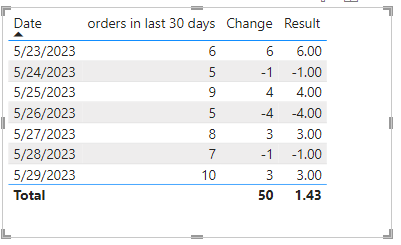cancel
Showing results for
Did you mean:Helper I

## avg change in last 7 days for orders

Hi evryone,

I need help with the calculation:

I heve this measure:

unique_outlet_order_30d = CALCULATE([distinct orders],DATESBETWEEN('Date_Created_Date'[Date],LASTDATE('Date_Created_Date'[Date])-30, LASTDATE('Date_Created_Date'[Date])))

I need to calculate the change between today and yesterday for the last 7 days and to do avarge about the score

for example:
 orders in last 30 days change 29/05/2023 10 3 28/05/2023 7 -1 27/05/2023 8 3 26/05/2023 5 -4 25/05/2023 9 4 24/05/2023 5 -1 23/05/2023 6 6 avg 1.4

there is a way to calculate it with a measure?
2 ACCEPTED SOLUTIONSSuper User

@sapirmarko , Try like

Calculate(AVERAGEX(Values('Date'[Date]), [This Day] - [diff]),DATESINPERIOD('Date'[Date ],MAX('Date'[Date ]),-7,DAY) )Community Support

Hi, @sapirmarko

You can try the following methods.

Measure:

``````Change =
Var _Previousdate=MAXX(FILTER(ALL('Table'),[Date]<SELECTEDVALUE('Table'[Date])),[Date])
Var _Previousorder=CALCULATE(SUM('Table'[orders in last 30 days]),FILTER(ALL('Table'),[Date]=_Previousdate))
Var _Currentorder=SUM('Table'[orders in last 30 days])
Return
_Currentorder-_Previousorder``````
``Result = IF(HASONEVALUE('Table'[Date]),[Change],AVERAGEX('Table',[Change]))``Is this the result you expect?

Best Regards,

Community Support Team _Charlotte

If this post helps, then please consider Accept it as the solution to help the other members find it more quickly.

3 REPLIES 3Helper I

Thanks! it's working great!Community Support

Hi, @sapirmarko

You can try the following methods.

Measure:

``````Change =
Var _Previousdate=MAXX(FILTER(ALL('Table'),[Date]<SELECTEDVALUE('Table'[Date])),[Date])
Var _Previousorder=CALCULATE(SUM('Table'[orders in last 30 days]),FILTER(ALL('Table'),[Date]=_Previousdate))
Var _Currentorder=SUM('Table'[orders in last 30 days])
Return
_Currentorder-_Previousorder``````
``Result = IF(HASONEVALUE('Table'[Date]),[Change],AVERAGEX('Table',[Change]))``Is this the result you expect?

Best Regards,

Community Support Team _Charlotte

If this post helps, then please consider Accept it as the solution to help the other members find it more quickly.Super User

@sapirmarko , Try like

Calculate(AVERAGEX(Values('Date'[Date]), [This Day] - [diff]),DATESINPERIOD('Date'[Date ],MAX('Date'[Date ]),-7,DAY) )